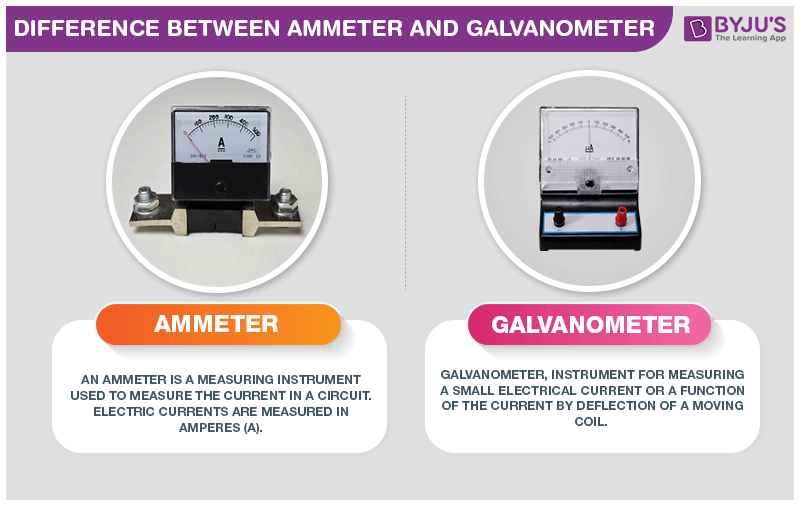# Difference Between Ammeter and Galvanometer

The major significant difference between ammeter and galvanometer is that ammeter shows only the magnitude of the current. Whereas, the galvanometer shows both the direction and magnitude of the current.## Difference Between Ammeter and Galvanometer

The galvanometer deflects the flow of current with the help of a moving coil which rotates between the permanent magnets and with the flow of current, deflection of the coil occurs. A galvanometer can be turned into an ammeter by parallely connecting a resistance in the electric circuit. It can be used as a voltmeter when resistance is connected in series with it.

Ammeter is used to measure the magnitude of current. It is also called as ampere meter because ampere is the unit of current.

Difference Between Ammeter and Galvanometer
Ammeter Galvanometer
It is a device used to determine the magnitude of current flowing in a circuit. It is a device used to detect the strength and direction of small current present in the circuit
It works with or without the presence of the magnetic field. It works due to the presence of the magnetic field
It measures both the Alternate and Direct current It measures only the direct current
It is less sensitive It is more sensitive
It is more accurate It is less accurate
It could be a mechanical or electronic device It is a mechanical device
It is used in an electrical circuit It is used in bridge and potentiometer

To learn about galvanometer to ammeter conversion, click on the video below.These were some difference between ammeter and galvanometer. If you wish to find out more, download BYJU’S – The Learning App.

RELATED ARTICLES:

## Frequently Asked Questions – FAQs

### How a galvanometer can be used as an ammeter?

A galvanometer is used for measuring or for detecting small currents. But by converting a galvanometer into an ammeter large currents can be detected. To convert a galvanometer into an ammeter, a low resistance known as shunt resistance is connected parallel to the galvanometer.

### What is a shunt resistance?

A resistor with a low value of resistance is known as shunt resistance. The material used in the shunt resistor is made from using a low-temperature coefficient resistance. When it is connected in parallel with an ammeter, it can be used for measuring the range to which it is extended. When the same shunt resistance is connected in series with the load, then the current through the load can be determined. Shunt resistance is used for converting a galvanometer into an ammeter.

### What is the reduction factor?

A reduction factor is used in a tangent galvanometer which is equal to the current which is required to pass through the galvanometer so that there is a production of 45o deflection. The reduction factor is denoted by the letter K and is measured in ampere.

### Why the resistance of an ammeter kept low?

The resistance of an ammeter is kept low because when the resistance range is kept at high values, the current passing through the circuit will decrease. Therefore, it is kept at low values.

### Why is an ammeter always connected in series?

An ammeter is always connected in series because the internal resistance of an ammeter is low. If the ammeter is connected in parallel, there are high chances of short circuit.

Test your knowledge on Ammeter and galvanometer difference

#### 1 Comment

1. Stan BTS

That was helpful! It solved my doubt